Function Repository Resource:

# GlaucomaRisk

Compute probability of having glaucoma given the intraocular pressure

 ResourceFunction["GlaucomaRisk"][p] returns the probability of having glaucoma given the intraocular pressure p.

## Details

p should be a nonnegative real number, a String or a Quantity.
If p is a number with no unit, millimeters of mercury is taken as the pressure unit.
If p is a String, the corresponding pressure unit should be provided, e.g. "10 mmHg".
p can be given as a Quantity whose unit is a pressure unit.
The result of ResourceFunction["GlaucomaRisk"] is returned as a percentage.
Intraocular pressure is the only modifiable variable used to prevent or delay progression of glaucoma.

## Examples

### Basic Examples (3)

Find the glaucoma risk corresponding to a given intraocular pressure, assuming units in millimeters of mercury:

 In:=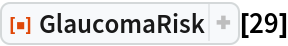Out=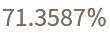Obtain the glaucoma risk for an intraocular pressure given as a String:

 In:=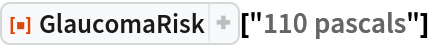Out=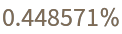In:=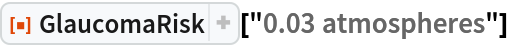Out=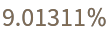Find the glaucoma risk for an intraocular pressure specified as a quantity in units of pascals:

 In:=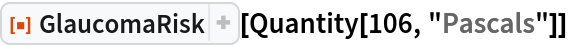Out=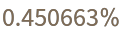### Neat Examples (1)

Calculate the probability of having glaucoma given the intraocular pressure:

 In:=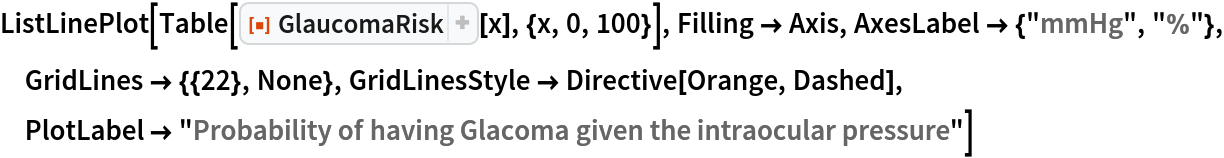Out=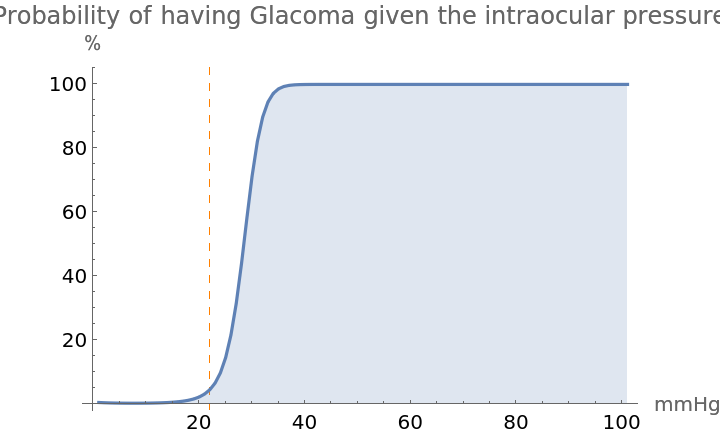## Publisher

WolframSpecialProjects

## Version History

• 1.0.0 – 06 January 2021